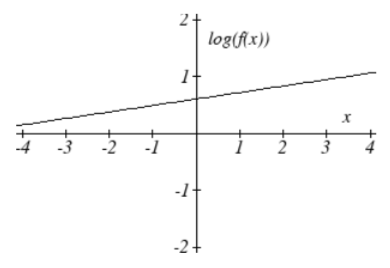$$\newcommand{\id}{\mathrm{id}}$$ $$\newcommand{\Span}{\mathrm{span}}$$ $$\newcommand{\kernel}{\mathrm{null}\,}$$ $$\newcommand{\range}{\mathrm{range}\,}$$ $$\newcommand{\RealPart}{\mathrm{Re}}$$ $$\newcommand{\ImaginaryPart}{\mathrm{Im}}$$ $$\newcommand{\Argument}{\mathrm{Arg}}$$ $$\newcommand{\norm}{\| #1 \|}$$ $$\newcommand{\inner}{\langle #1, #2 \rangle}$$ $$\newcommand{\Span}{\mathrm{span}}$$

# 4.7.7E: Fitting Exponential Models to Data (Exercises)

$$\newcommand{\vecs}{\overset { \rightharpoonup} {\mathbf{#1}} }$$ $$\newcommand{\vecd}{\overset{-\!-\!\rightharpoonup}{\vphantom{a}\smash {#1}}}$$$$\newcommand{\id}{\mathrm{id}}$$ $$\newcommand{\Span}{\mathrm{span}}$$ $$\newcommand{\kernel}{\mathrm{null}\,}$$ $$\newcommand{\range}{\mathrm{range}\,}$$ $$\newcommand{\RealPart}{\mathrm{Re}}$$ $$\newcommand{\ImaginaryPart}{\mathrm{Im}}$$ $$\newcommand{\Argument}{\mathrm{Arg}}$$ $$\newcommand{\norm}{\| #1 \|}$$ $$\newcommand{\inner}{\langle #1, #2 \rangle}$$ $$\newcommand{\Span}{\mathrm{span}}$$ $$\newcommand{\id}{\mathrm{id}}$$ $$\newcommand{\Span}{\mathrm{span}}$$ $$\newcommand{\kernel}{\mathrm{null}\,}$$ $$\newcommand{\range}{\mathrm{range}\,}$$ $$\newcommand{\RealPart}{\mathrm{Re}}$$ $$\newcommand{\ImaginaryPart}{\mathrm{Im}}$$ $$\newcommand{\Argument}{\mathrm{Arg}}$$ $$\newcommand{\norm}{\| #1 \|}$$ $$\newcommand{\inner}{\langle #1, #2 \rangle}$$ $$\newcommand{\Span}{\mathrm{span}}$$

section 4.7 exercise

Graph each function on a semi-log scale, then find a formula for the linearized function in the form $$\log \left(f\left(x\right)\right)=mx+b$$.

1. $$f\left(x\right)=4\left(1.3\right)^{x}$$

2. $$f\left(x\right)=2\left(1.5\right)^{x}$$

3. $$f\left(x\right)=10\left(0.2\right)^{x}$$

4. $$f\left(x\right)=30\left(0.7\right)^{x}$$

The graph below is on a semi-log scale, as indicated. Find a formula for the exponential function $$y(x)$$.

5.6.7.8.Use regression to find an exponential function that best fits the data given.13. Total expenditures (in billions of dollars) in the US for nursing home care are shown below. Use regression to find an exponential function that models the data. What does the model predict expenditures will be in 2015?

 Year 1990 1995 2000 2003 2005 2008 Expenditure 53 74 95 110 121 138

14. Light intensity as it passes through water decreases exponentially with depth. The data below shows the light intensity (in lumens) at various depths. Use regression to find an function that models the data. What does the model predict the intensity will be at 25 feet?

 Depth (ft) 3 6 9 12 15 18 Lumen 11.5 8.6 6.7 5.2 3.8 2.9

15. The average price of electricity (in cents per kilowatt hour) from 1990 through 2008 is given below. Determine if a linear or exponential model better fits the data, and use the better model to predict the price of electricity in 2014.

 Year 1986 1988 1990 1995 1997 2000 2002 2004 2006 2008 Cost 7.83 8.21 8.38 8.36 8.26 8.24 8.44 8.95 10.4 11.26

16. The average cost of a loaf of white bread from 1986 through 2008 is given below. Determine if a linear or exponential model better fits the data, and use the better model to predict the price of a loaf of bread in 2016.

 Year 1986 1988 1990 1995 1997 2000 2002 2004 2006 2008 Cost 0.57 0.66 0.7 0.84 0.88 0.99 1.03 0.97 1.14 1.42

1. $$\text{log} (f(x)) = \text{log} (1.3)x + \text{log} (4)$$3. $$\text{log} (f(x)) = \text{log} (0.2) x + 1$$5. $$y = e^{\dfrac{1}{2}x - 1} = e^{-1} e^{\dfrac{1}{2}x} \approx 0.368 (1.6487)^x$$

7. $$y = 10^{-x - 2}. = 10^{-2} 10^{-1x} = 0.01 (0.1)^x$$

9. $$y = 776.682 (1.426)^x$$

11. $$y = 731.92(0.738)^x$$

13. Expenditures are approximately \$205

15. $$y = 7.599(1.016)^x$$ $$r = 0.83064$$, $$y = 0,1493x + 7.4893$$, $$r = 0.81713$$. Using the better function, we predict electricity will be 11.157 cents per kwh